Fluid DynamicsNext: Cellular Automata Up: No Title Previous: Basic Concepts of Traffic

# Fluid Dynamics

Fluid-dynamic approaches have been developed since the 1950's . The road traffic is modeled as a one-dimensional compressible flow with density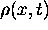with velocity v(x,t). The continuity equation reads,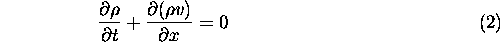The dynamics can be expressed by the Navier-Stokes equation , which states that the particle density times acceleration equals the sum of the acting forces,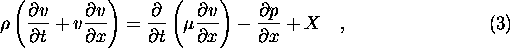where p is the local pressure of the vehicular gas,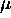is the viscosity and X represents the force caused by the interaction of the individual vehicles. The forces are derived from the density dependence of the velocity given by the fundamental diagram such as shown in Fig. 2,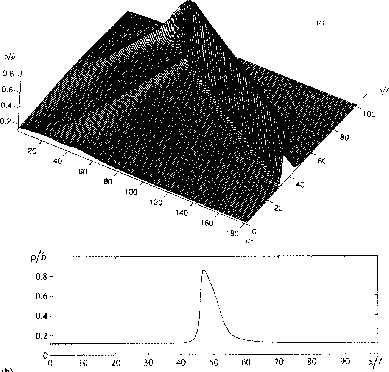Figure 3: Time evolution of a traffic jam showing the densityupper graph and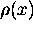for a particular time (taken from ).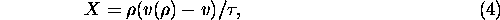where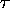is the relaxation time of the process. Kerner el al. [3, 4, 5] showed that this ansatz leads to the formation of stationary clusters (jams). Their form is shown in Fig. 3. The model was significantly improved by Helbing  who introduced the variance of the velocities as an additional space and time dependent variable. He showed the this approach leads to a more realistic behavior when jams develop.

Burkhard Militzer
Sat May 9 11:34:31 CDT 1998